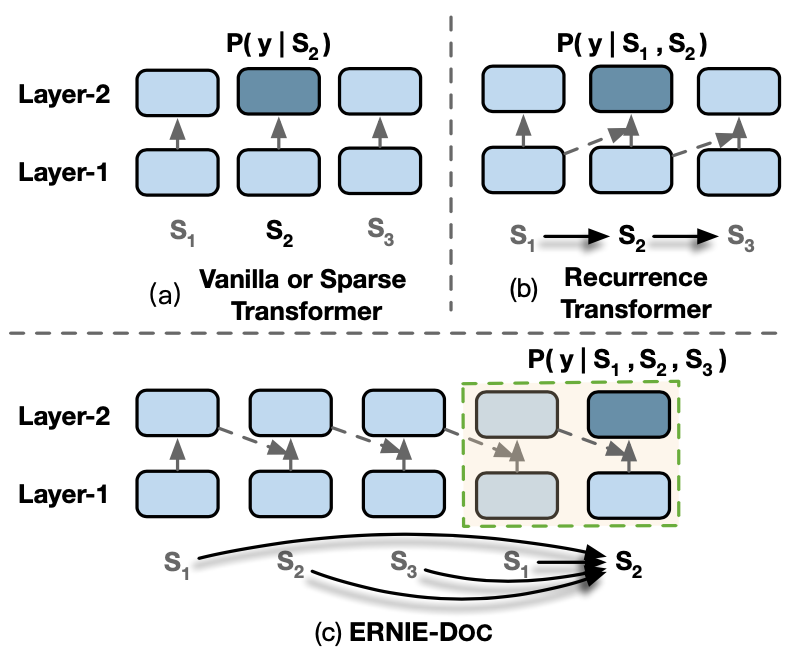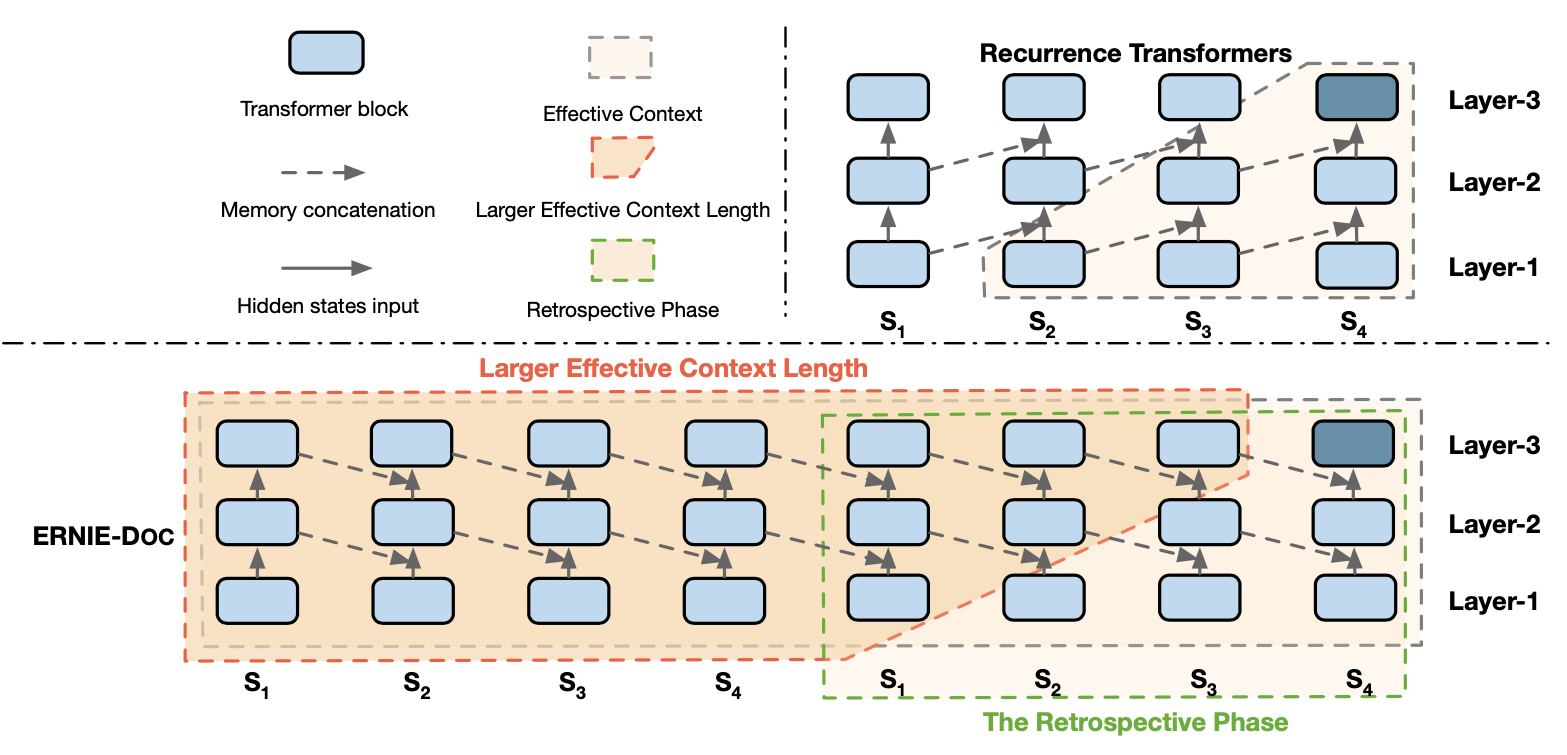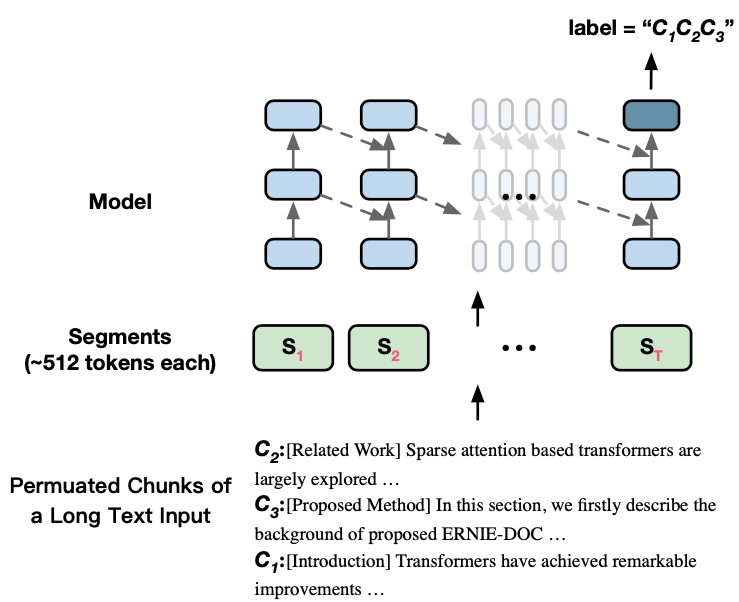# ERNIE-Doc: A Retrospective Long-Document Modeling Transformer¶

## 1. ERNIE-Doc的由来¶• Retrospective feed mechanism: 将文本两次传入模型获得文本序列的representation，第一次将获得完整的文本序列表示，然后该序列的representation继续参与第二次的编码过程，这样该文本序列在第二次编码过程中，每个token位置便能获得序列完整的双向信息。

• Enhanced recurrence mechanism: 使用了一种增强的Segment循环机制进行建模。

• Segment-reordering objective: 对一篇文档中的各个segment随机打乱，获得多个乱序文档，然后要求模型预测这些文档中哪个是正常语序的文档。

## 2. 经典/Recurrence Transformer的计算¶

\begin{split} \begin{align} & \tilde{h}_{\tau+1}^{n-1} = \left\{ \begin{matrix} h_{\tau+1}^{n-1}, \qquad \text{Vanilla Transformers} \\ \left[ \text{SG}(h_{\tau}^{n-1}) \; \circ \;h_{\tau+1}^{n-1} \right], \qquad \text{Recurrence Transformers} \end{matrix} \right. \\ & q_{\tau+1}^{n}, \; k_{\tau+1}^n, \; v_{\tau+1}^n = h_{\tau+1}^{n-1}W_{q}^{\mathrm{ T }}, \; \tilde{h}_{\tau+1}^{n-1}W_{k}^{\mathrm{ T }}, \; \tilde{h}_{\tau+1}^{n-1}W_{v}^{\mathrm{ T }} \\ & h_{\tau+1}^n = \text{Transformer-Layer}(q_{\tau+1}^{n}, \; k_{\tau+1}^n, \; v_{\tau+1}^n) \end{align} \end{split}

## 3. Retrospective feed mechanism¶

ERNIE-Doc借鉴了人类阅读的行为习惯，在人类阅读时会分为两个阶段：首先会快速略读一下文档内容，然后回过头来仔细阅读。ERNIE-Doc基于此设计了Retrospective feed mechanism，该机制同样包含两个阶段：Skimming phase 和 Retrospective phase。

\begin{split} \begin{align} \hat{H} &= \left[ \hat{H}_{1:T}^{1} \circ \hat{H}_{1:T}^{2} \cdot \cdot \cdot \circ \; \hat{H}_{1:T}^N \right], \quad \text{(skimming phase)} \\ \hat{H}_{1:T}^{i} &= \left[ \hat{h}_1^i \circ \hat{h}_2^i \cdot \cdot \cdot \circ \;\hat{h}_T^i \right] , \quad \text{(skimming phase)} \\ \tilde{h}_{\tau+1}^{n-1} &= \left[{SG}(\hat{H }\circ h_{\tau}^{n-1}) \circ h_{\tau+1}^{n-1} \right], \quad \text{(retrospective phase)} \end{align} \end{split}

• $$T$$​：一篇文档的Segment数量； $$N$$：Transformer模型层的数量；$$L$$​：每个Segment中的最大token数量。

• $$\hat{H} \in \mathbb{R}^{(L \times T \times N) \times d}$$​​​​​：一篇文档在所有层中输出的编码向量。

• $$\hat{H}_{1:T}^i \in \mathbb{R}^{(L \times T) \times d}$$: 一篇文档在第$$i$$​层产生的编码向量。

• $$\hat{h}_\tau^i$$：第$$\tau$$个Segment在第$$i$$层产生的编码向量。

## 4. Enhanced Recurrence Mechanism¶

ERNIE-Doc通过使用Retrospective feed mechanism和Enhanced Recurrence Mechanism两种方式，增大了计算每个segment时的有效上下文长度。但是第3节引入的公式计算复杂度是很高，因此 Enhanced Recurrence Mechanism期望前一个Segment便能获得完整的文档信息，然后直接融入前一个Segment便能使得当前Segment计算融入完整的文档信息。

$\tilde{h}_{\tau+1}^{n-1} = \left[{SG}(h_\tau^n) \circ h_{\tau+1}^{n-1} \right]$## 5. Segment-Reordering Objective¶$\underset {\theta}{\text{max}} \; \text{log} \; p_\theta(S_\tau|\hat{S}_\tau) + \mathbb{1}_{\tau=T}\; \text{log}\;p_\theta(D|\hat{D})$